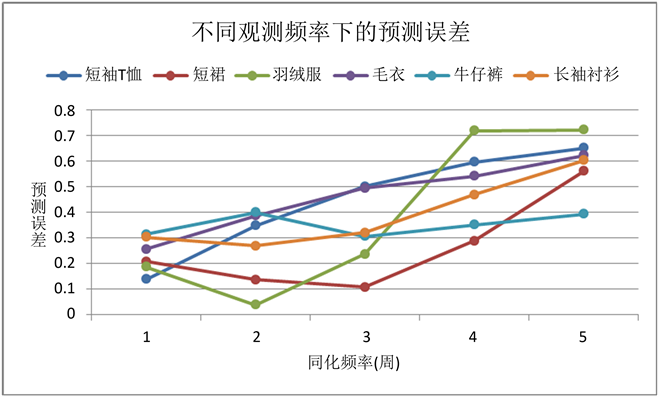﻿ 基于需求学习的多阶段动态折扣和库存优化研究——以服装销售为例

# 基于需求学习的多阶段动态折扣和库存优化研究——以服装销售为例 Multi-Stage Dynamic Discount and Inventory Optimization Model Based on Demand Learning: Taking Garment Sales as an Example

Abstract: Aiming at the uncertainties of demand model, based on the known prior demand model, data assimilation technology is applied to continuously learn the parameters of demand model, and the state-valued demand parameters of the model are optimized by using the data of each observation time. A multi-stage discount and inventory model based on demand learning is established to formulate a reasonable discount strategy to meet the business sales objectives. Empirical research shows that the proposed model can effectively reduce the inventory level of merchants by making reasonable discounts under demand uncertainty, and provide scientific basis for discount promotion. Through sensitivity analysis of initial inventory and initial price, the optimal initial inventory and initial price can be found. It provides strong support for the determination of order quantity and initial pricing, and improves the control level of dynamic system.

1. 引言

2. 模型建立

2.1. 算法介绍

1) 预测。令系统状态向量 $\theta ~N\left({\theta }_{0},{\sigma }^{2}{P}_{0}\right)$ ，那么状态预测为 ${\theta }_{n}=A{\theta }_{n-1}+\omega$

2) 计算卡尔曼增益 ${k}_{n}$ 。根据第n阶段的观测数据得到测量矩阵 ${H}_{n}$ 和测量噪声V，其中R是V的协方差，那么 ${k}_{n}={P}_{n}{{H}^{\prime }}_{n}/\left({H}_{n}{P}_{n}{{H}^{\prime }}_{n}+R\right)$

3) 计算最优估计值。根据卡尔曼增益 ${k}_{n}$ 得到现在阶段n的最优化参数估算值： ${{\theta }^{\prime }}_{n}={\theta }_{n}+{k}_{n}\left({z}_{n}-{H}_{n}{\theta }_{n}\right)$ ，其中 ${z}_{n}={H}_{n}{\theta }_{n}+V$ ，为第n阶段的系统测量值；

4) 更新。为了要卡尔曼滤波器不断的运行下去直到系统过程结束，还要更新第n阶段下 ${\theta }_{n}$ 的协方差矩阵 ${{P}^{\prime }}_{n}$ ，计算公式为 ${{P}^{\prime }}_{n}=\left(1-{k}_{n}{H}_{n}\right){P}_{n}$

2.2. 基于参数更新的动态折扣和库存优化模型

2.2.1. 动态折扣和库存优化模型建立

${I}_{n+1}={I}_{n}-{Q}_{n}={I}_{0}-{\sum }_{1}^{n}{Q}_{n}$ (1)

${Q}_{n}={\int }_{0}^{{t}_{n}}{q}_{n}\left(t\right)\text{d}t={q}_{n}{t}_{n}={\text{e}}^{\alpha +\beta {\gamma }_{n}+ϵ}{t}_{n}=M{\text{e}}^{\alpha +\beta {\gamma }_{n}}{t}_{n}$ (2)

${H}_{n}={\int }_{0}^{{t}_{n}}{I}_{n}\left(t\right)h\text{d}t={I}_{n}{t}_{n}h-\frac{{q}_{n}{t}_{n}\left(1+{t}_{n}\right)}{2}h$ (3)

${F}_{n}={Q}_{n}{p}_{0}{\gamma }_{n}=M{\text{e}}^{\alpha +\beta {\gamma }_{n}}{t}_{n}{p}_{0}{\gamma }_{n}$ (4)

${R}_{n}={F}_{n}-{H}_{n}-c{Q}_{n}=M{\text{e}}^{\alpha +\beta {\gamma }_{n}}{t}_{n}\left({p}_{0}{\gamma }_{n}-c\right)-{I}_{n}{t}_{n}h+\frac{M{\text{e}}^{\alpha +\beta {\gamma }_{n}}{t}_{n}\left(1+{t}_{n}\right)}{2}h$ (5)

$\begin{array}{l}\mathrm{max}{\sum }_{n=1}^{n=m}{R}_{n}\\ \text{s}\text{.t}:{I}_{m+1}=0\end{array}$ (6)

2.2.2. 最优折扣求解

${f}_{n}\left({I}_{n}\right)=\mathrm{max}\left\{{R}_{n}+{f}_{n+1}\left({I}_{n+1}\right)\right\}$ (7)

${f}_{m+1}\left({I}_{m=1}\right)=0,\text{\hspace{0.17em}}\text{\hspace{0.17em}}n=m,m-1,\cdots ,1$ (8)

1) 2阶段模型求解

$m=2$ 时，模型目标为： $\text{max}\left(E\left({R}_{1}\right)+E\left({R}_{2}\right)\right)$ ，先验信息 $\theta ={\left(\alpha ,\beta \right)}^{\prime }~N\left({\theta }_{0},{\sigma }^{2}{P}_{0}\right)$ ，可以通过之前的历史数据得到，根据卡尔曼滤波重新更新得到 ${\theta }_{1}$ ，以此类推，首先考虑第2阶段，进行 ${f}_{2}\left({I}_{2}\right)$ 的极值求解：

${f}_{2}\left({I}_{2}\right)={R}_{2}=M{\text{e}}^{\alpha +\beta {\gamma }_{2}}{t}_{2}\left({p}_{0}{\gamma }_{2}-c\right)-{I}_{2}{t}_{2}h+\frac{M{\text{e}}^{\alpha +\beta {\gamma }_{2}}{t}_{2}\left(1+{t}_{2}\right)}{2}h$ (9)

${\gamma }_{2}^{\ast }=\frac{\mathrm{ln}\left(\frac{{I}_{2}}{M{t}_{2}}\right)-{\alpha }_{1}}{{\beta }_{1}}$ (10)

$E\left({R}_{2}^{\ast }\right)={f}_{2}\left({I}_{2}\right)={I}_{2}\left({p}_{0}\frac{\mathrm{ln}\left(\frac{{I}_{2}}{M{t}_{2}}\right)-{\alpha }_{1}}{{\beta }_{1}}-c\right)-{I}_{2}{t}_{2}h+\frac{{I}_{2}\left(1+{t}_{2}\right)}{2}h$ (11)(12)

${\gamma }_{1}^{\ast }=\frac{\mathrm{ln}\left(\frac{{I}_{1}}{M\left({t}_{2}{\text{e}}^{\frac{{\beta }_{1}}{{\beta }_{0}}+\frac{2+{t}_{1}-{t}_{2}}{2{p}_{0}}h{\beta }_{1}-{t}_{2}M}+{t}_{1}\right)}\right)-{\alpha }_{0}}{{\beta }_{0}}$ (13)

2) 多阶段模型求解

$m>2$ 时，参数更新下的动态规划求解相当困难。但是基于上述动态规划求解过程可以采取一个近似定价策略：要找到每个阶段的最优折扣 ${\gamma }_{n}^{\ast }$ ，就是要下式最大化：

$\begin{array}{l}E\left({R}_{n}\right)=M{\text{e}}^{{\alpha }_{n-1}+{\beta }_{n-1}{\gamma }_{n}}{t}_{n}\left({p}_{0}{\gamma }_{n}-c\right)-{I}_{n}{t}_{n}h\\ \text{\hspace{0.17em}}\text{\hspace{0.17em}}\text{\hspace{0.17em}}\text{\hspace{0.17em}}\text{\hspace{0.17em}}\text{\hspace{0.17em}}\text{\hspace{0.17em}}\text{\hspace{0.17em}}\text{\hspace{0.17em}}\text{\hspace{0.17em}}\text{\hspace{0.17em}}+\frac{M{\text{e}}^{{\alpha }_{n-1}+{\beta }_{n-1}{\gamma }_{n}}{t}_{n}\left(1+{t}_{n}\right)}{2}h+\frac{1}{2}\frac{M{\text{e}}^{{\alpha }_{n}-1}}{{\beta }_{n}{}^{3}}{\sigma }_{{\beta }_{n}}^{2}{\sigma }_{{\alpha }_{n}}^{2}\end{array}$

${\gamma }_{n}^{\ast }=\frac{\mathrm{ln}\left(\frac{{I}_{n}}{M\left({t}_{n+1}{\text{e}}^{\frac{{\beta }_{n}}{{\beta }_{n-1}}+\frac{2+{t}_{n}-{t}_{n+1}}{2{p}_{0}}h{\beta }_{n}-{t}_{n+1}M}+{t}_{n}\right)}\right)-{\alpha }_{n-1}}{{\beta }_{n-1}}$ (14)

3. 实证分析

3.1. 数据源

3.2. 预测误差分析

$\text{Error}=\left({{\theta }^{\prime }}_{n}-{\theta }_{n}\right)/{\theta }_{n}$ (15)

3.3. 最优折扣策略计算

$M=E\left({\text{e}}^{{ϵ}_{t}}\right)={\text{e}}^{{\sigma }^{2}/2}=1.046$ ，参数更新频率为3周，即 ${t}_{n}=21$ 天，根据表1的各阶段参数的滤波估计值，计算最优折扣策略，期望销量和期望收益额(元)，结果如表2所示。Figure 1. Comparison of prediction errors under different assimilation frequenciesTable 2. Optimal discount strategy and inventory change in each stage

4. 参数灵敏度分析Table 3. Sensitivity analysis of initial inventoryTable 4. Sensitivity analysis of initial price

5. 结论

 Golabi, K. (1985) Optimal Inventory Policies When Ordering Prices Are Random. Operations Research, 33, 575-588.
https://doi.org/10.1287/opre.33.3.575

 Bitran, G.R. and Mondschein, S.V. (1997) Periodic Pricing of Seasonal Products in Retailing. Management Science, 43, 64-79.
https://doi.org/10.1287/mnsc.43.1.64

 Besbes, O. and Zeevi, A. (2009) Dynamic Pricing without Knowing the Demand Function: Risk Bounds and Near-Optimal Algorithms. Operations Research, 57, 1407-1420.
https://doi.org/10.1287/opre.1080.0640

 Araman, V.F. and Caldentey, R. (2009) Dynamic Pricing for Perishable Products with Demand Learning. Operations Research, 57, 1169-1188.
https://doi.org/10.1287/opre.1090.0725

 Lin, K.Y. (2006) Dynamic Pricing with Real-Time Demand Learning. European Journal of Operational Research, 174, 522-538.
https://doi.org/10.1016/j.ejor.2005.01.041

 Huang, C.L. and Xin, L.I. (2006) Experiments of Soil Moisture Data Assimilation System Based on Ensemble Kalman Filter. Plateau Meteorology, 112, 888-900.

 Carvalho, A.X. and Puterman, M.L. (2003) Dynamic Pricing and Reinforcement Learning. International Joint Conference on Neural Networks, 4, 2916-2921.
https://doi.org/10.1109/IJCNN.2003.1224034

Top